# Measurement of prompt D-meson production in p-Pb collisions at $\sqrt{s_{\rm NN}}$ = 5.02 TeV

The $p_{\rm T}$-differential production cross sections of the prompt charmed mesons $D^0$, $D^+$, $D^{*+}$ and $D_{\rm s}^{+}$ and their charge conjugate in the rapidity interval $-0.96 < y_{\rm cms} < 0.04$ were measured in p-Pb collisions at a centre-of-mass energy $\sqrt{s_{\rm NN}} = 5.02$ TeV with the ALICE detector at the LHC. The nuclear modification factor $R_{\rm pPb}$, quantifying the D-meson yield in p-Pb collisions relative to the yield in pp collisions scaled by the number of binary nucleon-nucleon collisions, is compatible within the 15-20% uncertainties with unity in the transverse momentum interval $1 < p_{\rm T} < 24$ GeV/$c$. No significant difference among the $R_{\rm pPb}$ of the four D-meson species is observed. The results are described within uncertainties by theoretical calculations that include initial-state effects. The measurement adds experimental evidence that the modification of the momentum spectrum of D mesons observed in Pb-Pb collisions with respect to pp collisions is due to strong final-state effects induced by hot partonic matter.

Figures

## Figure 1

 $\pt$-differential inclusive production cross section of prompt $\Dzero$, $\Dplus$, $\Dstar$ and $\rm D^{+}_s$ mesons in $\pPb$ collisionsat $\sNN$=5.02 TeV. Statistical uncertainties (bars) and systematic uncertainties (boxes) are shown.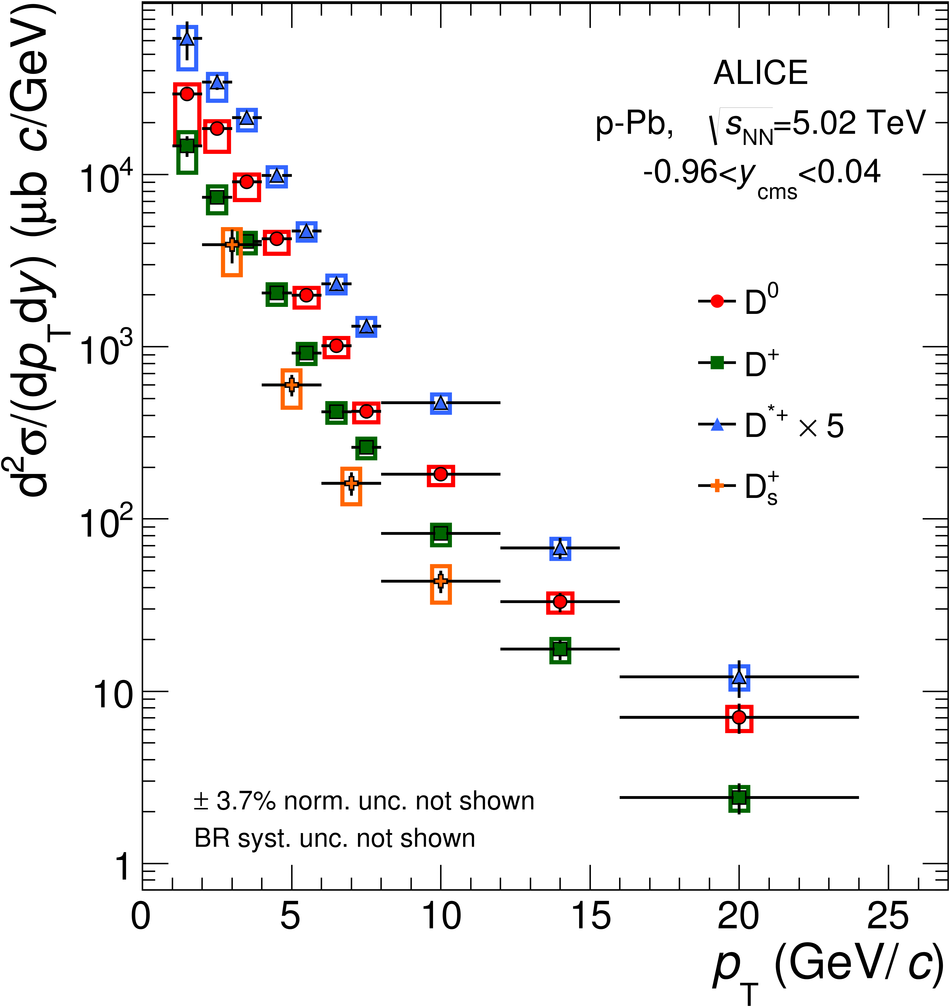## Figure 2

 $\RpPb$ as a function of $\pt$ for prompt $\Dzero$, $\Dplus$, $\Dstar$ and $\rm D^{+}_s$ mesons in $\pPb$ collisions at $\sNN$=5.02 TeV. Statistical (bars), systematic (empty boxes), and normalization (full box) uncertainties are shown.## Figure 3

 Average $\RpPb$ of prompt $\Dzero$, $\Dplus$and $\Dstar$ mesons as a function of $\pt$ compared to model calculations. Statistical (bars), systematic (empty boxes), and normalization (full box) uncertainties are shown.## Figure 4

 Average $\RpPb$ of prompt $\Dzero$, $\Dplus$ and $\Dstar$ mesons as a function of $\pt$ compared to D-meson $\Raa$ in the 20% most central and in the 40-80% Pb-Pb collisions at $\sNN$=2.76 TeV. Statistical (bars), systematic (empty boxes), and normalization (full boxes) uncertainties are shown.## Figure 1

 Invariant mass distributions of $\Dzero$ candidates and charge conjugates in four selected $\pt$ intervals considered for the analysis. The topological and kinematical selections applied to obtain these distributions are described in the paper. The fit functions showed in the figure consist of a sum of a Gaussian and an exponential to describe the signal and the background respectively. The values of the signal (S) and background counts (B) integrated in $\pm 3 \sigma$ region around the centroid of the Gaussian are reported.## Figure 2

 Invariant mass distributions of $\Dzero$ candidates and charge conjugates in the momentum interval $3< \pt< 4$~GeV/$c$.The values of the signal (S) and background counts (B) integrated in $\pm 3 \sigma$ region around the centroid of the Gaussian are reported.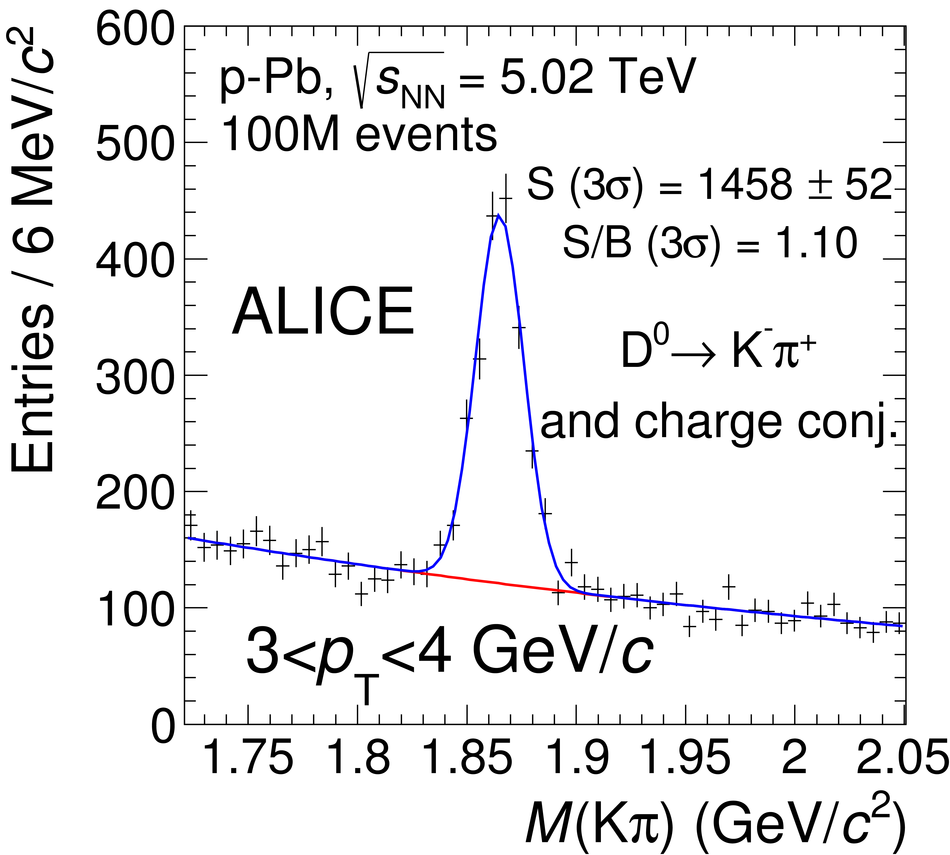## Figure 3

 Invariant mass distributions of $\Dplus$ candidates and charge conjugates in four selected $\pt$ intervals considered for the analysis. The topological and kinematical selections applied to obtain these distributions are described in the paper. The fit functions showed in the figure consist of a sum of a Gaussian and an exponential to describe the signal and the background respectively. The values of the signal (S) and background counts (B) integrated in $\pm 3 \sigma$ region around the centroid of the Gaussian are reported.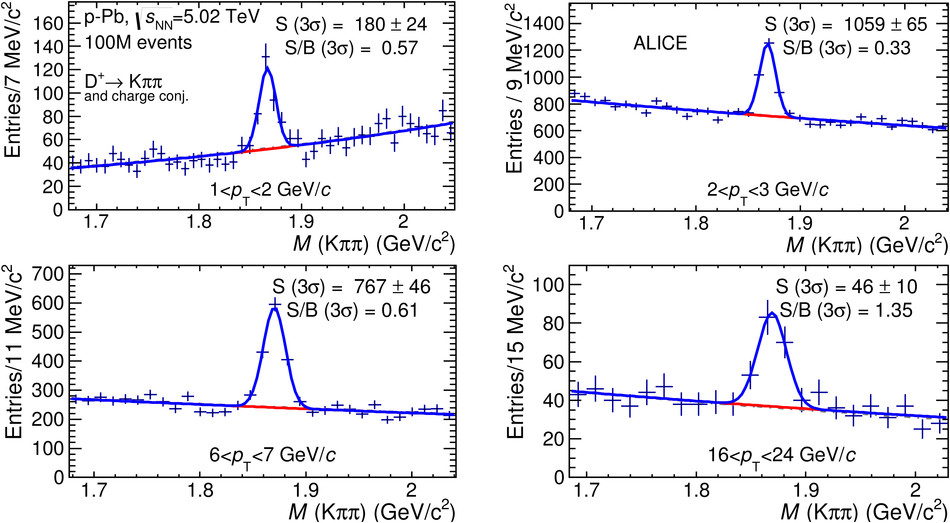## Figure 4

 Invariant mass distributions of $\Dplus$ candidates and charge conjugates in the momentum interval $3< \pt< 4$~GeV/$c$.The values of the signal (S) and background counts (B) integrated in $\pm 3 \sigma$ region around the centroid of the Gaussian are reported.## Figure 5

 Mass difference distributions of $\Dstar$ candidates and charge conjugates in four selected $\pt$ intervals considered for the analysis. The topological and kinematical selections applied to obtain these distributions are described in the paper. The fit functions showed in the figure consist of a sum of Gaussian and a threshold function ($a \sqrt{\Delta M - M_{\pi} } \cdot e^{b(\Delta M - M_{\pi})}$, where $M_{\pi}$ is the pion mass and $a$ and $b$ are free parameters) to describe the signal and the background respectively. The values of the signal (S) and background counts (B) integrated in $\pm 3 \sigma$ region around the centroid of the Gaussian are reported.## Figure 6

 Invariant mass distributions of $\Dstar$ candidates and charge conjugates in the momentum interval $3< \pt< 4$~GeV/$c$.The values of the signal (S) and background counts (B) integrated in $\pm 3 \sigma$ region around the centroid of the Gaussian are reported.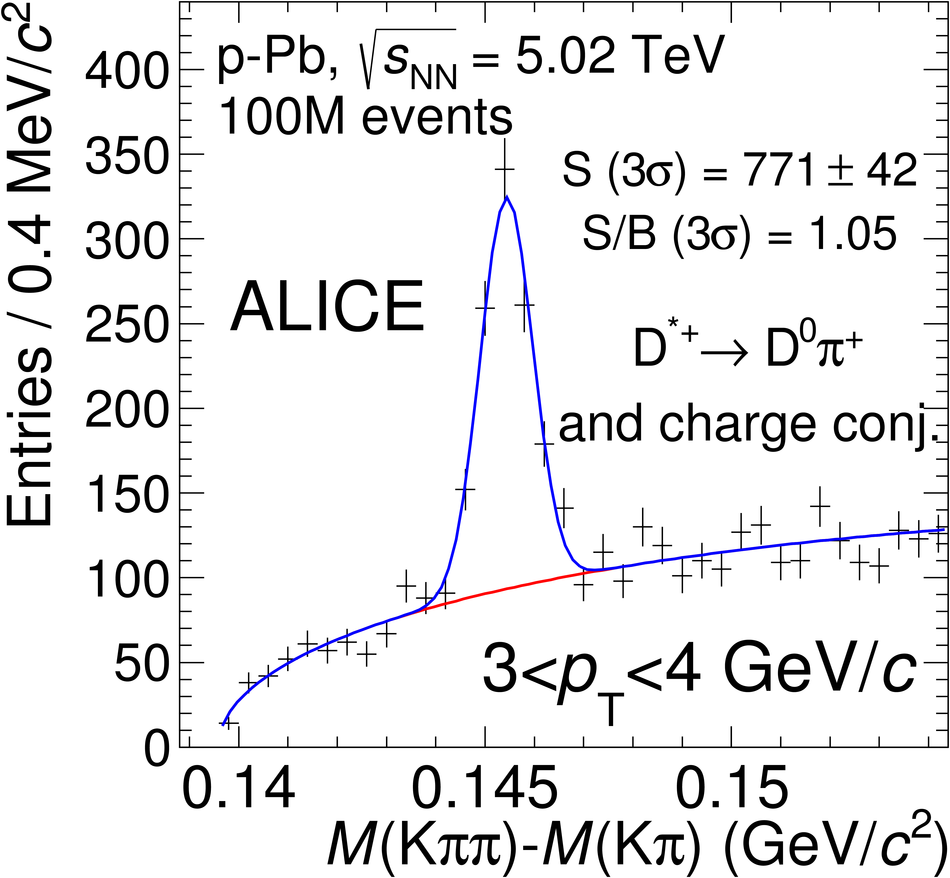## Figure 7

 Invariant mass distributions of $\rm D^+_s$ candidates and charge conjugates in the 4 $\pt$ intervals considered for the analysis. The topological and kinematical selections applied to obtain these distributions are described in the paper. The fit functions showed in the figure consist of a sum of a Gaussian and an exponential to describe the signal and the background respectively. The values of the signal (S) and background counts (B) integrated in $\pm 3 \sigma$ region around the centroid of the Gaussian are reported.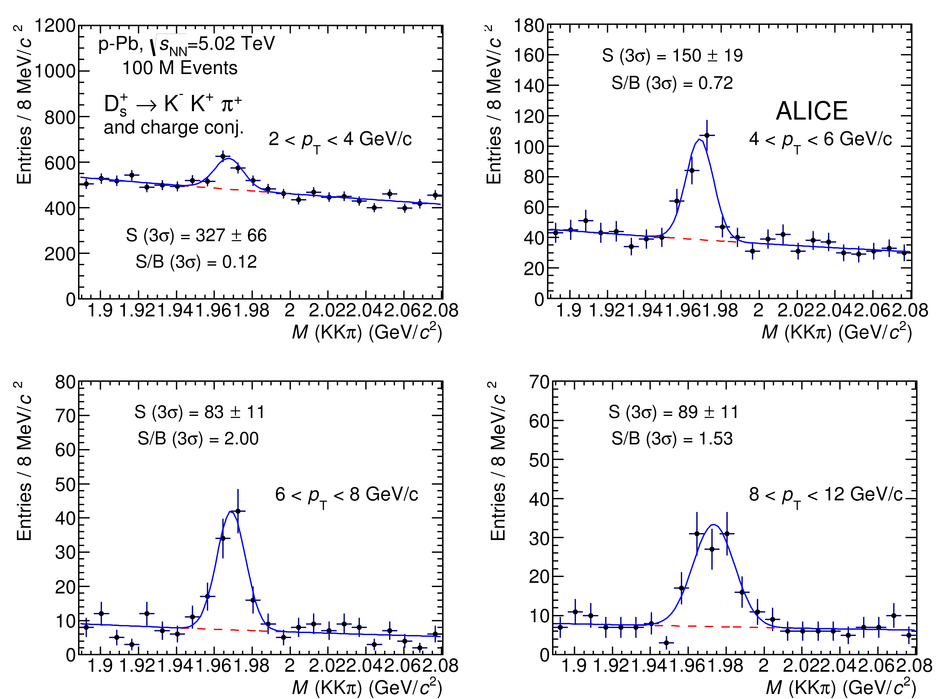## Figure 8

 Acceptance $\times$ efficiency corrections for prompt $\Dzero$, $\Dplus$, $\Dstar$ and $\rm D^+_s$ mesons (red empty circles) and for feed-down $\Dzero$, $\Dplus$, $\Dstar$ and $\rm D^+_s$ from B hadrons decays (blue empty squares) as a function of $\pt$. The efficiency without particle identification (filled green squares) is also shown for comparison.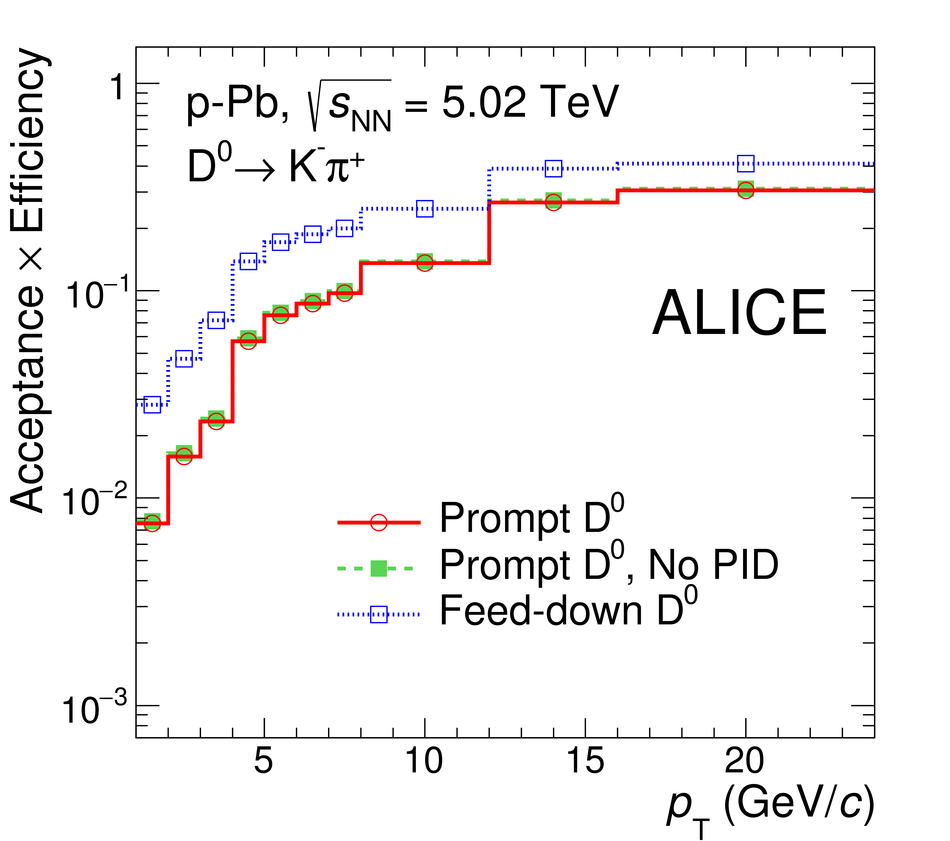## Figure 9

 $\pt$-differential production cross section of prompt $\Dzero$ mesons in $\pPb$ minimum-bias collisions at $\sqrt{s_{\mathrm{NN}}}=5.02$~TeV compared with a pp rescaled reference af the same center-of-mass $A \mathrm{d} \sigma / \mathrm{d} \pt$, where $A$ is the atomic mass number. Statistical uncertainties (bars) and systematic uncertainties from data analysis (empty boxes) and from feed-down subtraction (full boxes) are shown. Horizontal error bars reflect the bin widths, symbols are located at the center of the bin.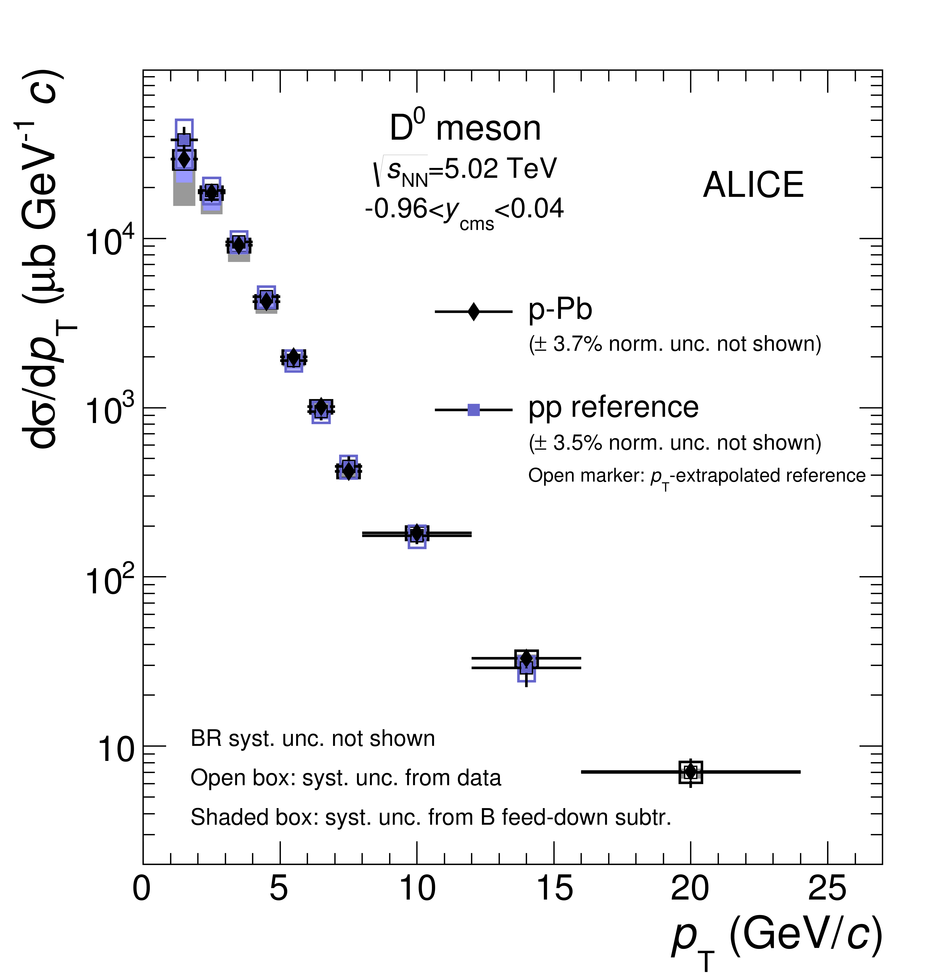## Figure 10

 $\pt$-differential production cross section of prompt $\Dplus$ mesons in $\pPb$ minimum-bias collisions at $\sqrt{s_{\mathrm{NN}}}=5.02$~TeV compared with a pp rescaled reference af the same center-of-mass $A \mathrm{d} \sigma / \mathrm{d} \pt$, where $A$ is the atomic mass number. Statistical uncertainties (bars) and systematic uncertainties from data analysis (empty boxes) and from feed-down subtraction (full boxes) are shown. Horizontal error bars reflect the bin widths, symbols are located at the center of the bin.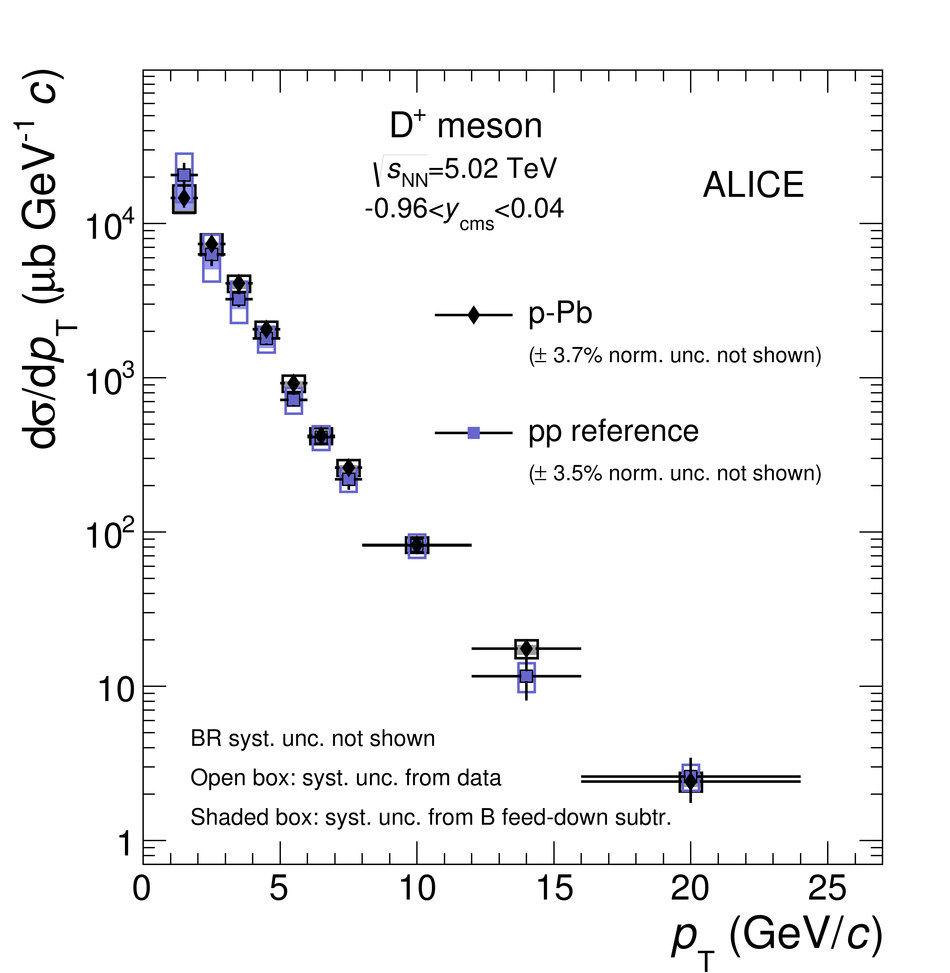## Figure 11

 $\pt$-differential production cross section of prompt $\Dstar$ mesons in $\pPb$ minimum-bias collisions at $\sqrt{s_{\mathrm{NN}}}=5.02$~TeV compared with a pp rescaled reference af the same center-of-mass $A \mathrm{d} \sigma / \mathrm{d} \pt$, where $A$ is the atomic mass number. Statistical uncertainties (bars) and systematic uncertainties from data analysis (empty boxes) and from feed-down subtraction (full boxes) are shown. Horizontal error bars reflect the bin widths, symbols are located at the center of the bin.## Figure 12

 $\pt$-differential production cross section of prompt $\rm D^+_s$ mesons in $\pPb$ minimum-bias collisions at $\sqrt{s_{\mathrm{NN}}}=5.02$~TeV compared with a pp rescaled reference af the same center-of-mass $A \mathrm{d} \sigma / \mathrm{d} \pt$, where $A$ is the atomic mass number. Statistical uncertainties (bars) and systematic uncertainties from data analysis (empty boxes) and from feed-down subtraction (full boxes) are shown. Horizontal error bars reflect the bin widths, symbols are located at the center of the bin.